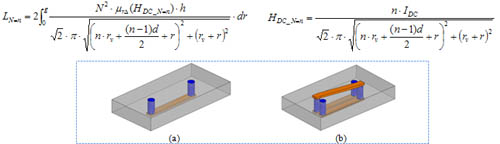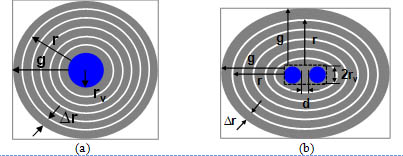RESEARCH

# Analytical Inductance Model for Planner Inductor with Non-uniform Flux PatternFig. 1. Planar inductor examples (a) 1-turn structure: N=1, (b) 2-turn structure: N=2.
In order to increase power density of POL converter, low profile planar inductor can be used as substrate for a 3D integrated converter to save footprint and fully utilize space. Fig.1 shows two examples of planar inductor. In Fig. 1, the 2-turn structure can be further extended to 3-turn and 4-turn structures. Because most planar inductors have non-uniform flux distribution, the conventional inductance calculation method, which assumes uniform flux distribution, cannot be used. Recently, CPES proposed a general analytical inductance model for planar inductor with non-uniform flux and permeability. With this method, the planar inductor is divided into many small concentric rings; the four corners of the core are ignored.

Fig. 2 shows the concept drawing of using this method on planar inductors shown in Fig.1. Because these inductors have symmetrical structure, Fig. 2 only shows half core. For different planar inductor structures, the shape of concentric ring is different. For single-turn structure (N=1), concentric rings have round shape; for multi-turn structure (N>1), concentric rings have ellipse shape. If the ring width is small enough, flux inside each ring can be assumed as constant. The permeability of each small ring is determined by DC pre-magnetization flux field intensity. The general equations for calculating inductance of these planar inductors are:Fig. 2. Concept drawing of dividing half core of planar inductor into many concentric rings (a) 1-turn structure: N=1, (b) 2-turn structure: N=2.
INDUSTRY PARTNERS